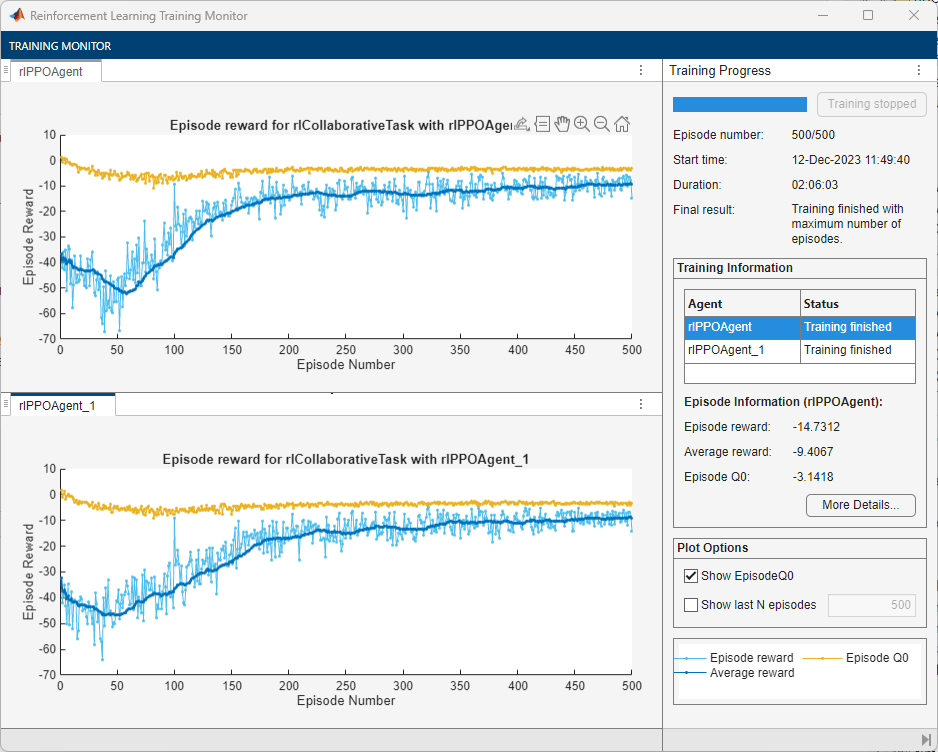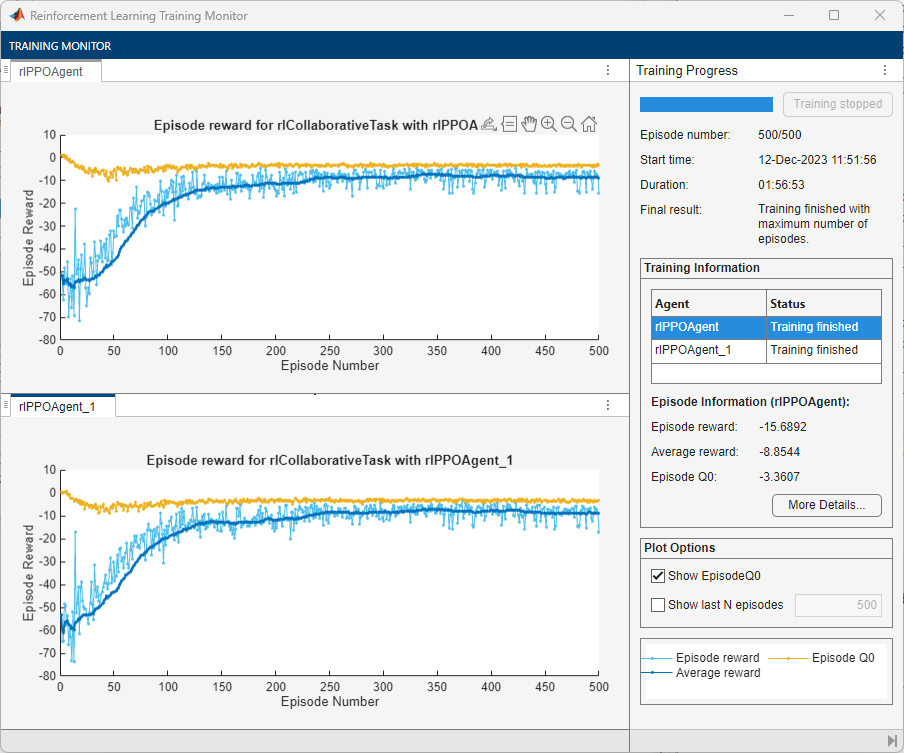# Train Multiple Agents to Perform Collaborative Task

This example shows how to set up a multi-agent training session on a Simulink® environment. In the example, you train two agents to collaboratively perform the task of moving an object.The environment in this example is a frictionless two dimensional surface containing elements represented by circles. A target object C is represented by the blue circle with a radius of 2 m, and robots A (red) and B (green) are represented by smaller circles with radii of 1 m each. The robots attempt to move object C outside a circular ring of a radius 8 m by applying forces through collision. All elements within the environment have mass and obey Newton's laws of motion. In addition, contact forces between the elements and the environment boundaries are modeled as spring and mass damper systems. The elements can move on the surface through the application of externally applied forces in the X and Y directions. There is no motion in the third dimension and the total energy of the system is conserved.

Set the random seed and create the set of parameters required for this example.

```rng(10) rlCollaborativeTaskParams```

```mdl = "rlCollaborativeTask"; open_system(mdl)```For this environment:

• The 2-dimensional space is bounded from –12 m to 12 m in both the X and Y directions.

• The contact spring stiffness and damping values are 100 N/m and 0.1 N/m/s, respectively.

• The agents share the same observations for positions, velocities of A, B, and C and the action values from the last time step.

• The simulation terminates when object C moves outside the circular ring.

• At each time step, the agents receive the following reward:

`$\begin{array}{l}{\mathit{r}}_{\mathit{A}}={\mathit{r}}_{\mathrm{global}}+{\mathit{r}}_{\mathrm{local},\mathit{A}}\\ {\mathit{r}}_{\mathit{B}}={\mathit{r}}_{\mathrm{global}}+{\mathit{r}}_{\mathrm{local},\mathit{B}}\\ {\mathit{r}}_{\mathrm{global}}=0.001{\mathit{d}}_{\mathit{c}}\\ {\mathit{r}}_{\mathrm{local},\mathit{A}}=-0.005{\mathit{d}}_{\mathrm{AC}}-0.008{\mathit{u}}_{\mathit{A}}^{2}\\ {\mathit{r}}_{\mathrm{local},\mathit{B}}=-0.005{\mathit{d}}_{\mathrm{BC}}-0.008{\mathit{u}}_{\mathit{B}}^{2}\end{array}$`

Here:

• ${\mathit{r}}_{\mathit{A}}$and ${\mathit{r}}_{\mathit{B}}$ are the rewards received by agents A and B, respectively.

• ${\mathit{r}}_{\mathrm{global}}$ is a team reward that is received by both agents as object C moves closer towards the boundary of the ring.

• ${\mathit{r}}_{\mathrm{local},\mathit{A}}$ and ${\mathit{r}}_{\mathrm{local},\mathit{B}}$ are local penalties received by agents A and B based on their distances from object C and the magnitude of the action from the last time step.

• ${\mathit{d}}_{\mathit{C}}$ is the distance of object C from the center of the ring.

• ${\mathit{d}}_{\mathrm{AC}}$ and ${\mathit{d}}_{\mathrm{BC}}$ are the distances between agent A and object C and agent B and object C, respectively.

• The agents apply external forces on the robots that result in motion. ${\mathit{u}}_{\mathit{A}}$ and ${\mathit{u}}_{\mathit{B}}$ are the action values of the two agents A and B from the last time step. The range of action values is between -1 and 1.

#### Environment

To create a multi-agent environment, specify the block paths of the agents using a string array. Also, specify the observation and action specification objects using cell arrays. The order of the specification objects in the cell array must match the order specified in the block path array. When agents are available in the MATLAB workspace at the time of environment creation, the observation and action specification arrays are optional. For more information on creating multi-agent environments, see `rlSimulinkEnv`.

Create the I/O specifications for the environment. In this example, the agents are homogeneous and have the same I/O specifications.

```% Number of observations numObs = 16; % Number of actions numAct = 2; % Maximum value of externally applied force (N) maxF = 1.0; % I/O specifications for each agent oinfo = rlNumericSpec([numObs,1]); ainfo = rlNumericSpec([numAct,1], ... UpperLimit= maxF, ... LowerLimit= -maxF); oinfo.Name = "observations"; ainfo.Name = "forces";```

```blks = ["rlCollaborativeTask/Agent A", "rlCollaborativeTask/Agent B"]; obsInfos = {oinfo,oinfo}; actInfos = {ainfo,ainfo}; env = rlSimulinkEnv(mdl,blks,obsInfos,actInfos);```

Specify a reset function for the environment. The reset function `resetRobots` ensures that the robots start from random initial positions at the beginning of each episode.

`env.ResetFcn = @(in) resetRobots(in,RA,RB,RC,boundaryR);`

#### Agents

This example uses two Proximal Policy Optimization (PPO) agents with continuous action spaces. The agents apply external forces on the robots that result in motion. To learn more about PPO agents, see Proximal Policy Optimization (PPO) Agents.

The agents collect experiences until the experience horizon (600 steps) is reached. After trajectory completion, the agents learn from mini-batches of 300 experiences. An objective function clip factor of 0.2 is used to improve training stability and a discount factor of 0.99 is used to encourage long-term rewards.

Specify the agent options for this example.

```agentOptions = rlPPOAgentOptions(... ExperienceHorizon=600,... ClipFactor=0.2,... EntropyLossWeight=0.01,... MiniBatchSize=300,... NumEpoch=4,... AdvantageEstimateMethod="gae",... GAEFactor=0.95,... SampleTime=Ts,... DiscountFactor=0.99); agentOptions.ActorOptimizerOptions.LearnRate = 1e-4; agentOptions.CriticOptimizerOptions.LearnRate = 1e-4;```

Create the agents using the default agent creation syntax. For more information see `rlPPOAgent`.

```agentA = rlPPOAgent(oinfo, ainfo, ... rlAgentInitializationOptions(NumHiddenUnit= 200), agentOptions); agentB = rlPPOAgent(oinfo, ainfo, ... rlAgentInitializationOptions(NumHiddenUnit= 200), agentOptions);```

#### Training

To train multiple agents, you can pass an array of agents to the `train` function. The order of agents in the array must match the order of agent block paths specified during environment creation. Doing so ensures that the agent objects are linked to their appropriate I/O interfaces in the environment.

You can train multiple agents in a decentralized or centralized manner. In decentralized training, agents collect their own set of experiences during the episodes and learn independently from those experiences. In centralized training, the agents share the collected experiences and learn from them together. The actor and critic functions are synchronized between the agents after trajectory completion.

To configure a multi-agent training, you can create agent groups and specify a learning strategy for each group through the `rlMultiAgentTrainingOptions` object. Each agent group may contain unique agent indices, and the learning strategy can be `"centralized"` or `"decentralized"`. For example, you can use the following command to configure training for three agent groups with different learning strategies. The agents with indices `[1,2]` and `[3,4]` learn in a centralized manner while agent `4` learns in a decentralized manner.

` opts = rlMultiAgentTrainingOptions(AgentGroups= {[1,2], 4, [3,5]}, LearningStrategy= ["centralized","decentralized","centralized"])`

For more information on multi-agent training, type `help rlMultiAgentTrainingOptions` in MATLAB.

You can perform decentralized or centralized training by running one of the following sections using the Run Section button.#### 1. Decentralized Training

To configure decentralized multi-agent training for this example:

• Automatically assign agent groups using the `AgentGroups=auto` option. This allocates each agent in a separate group.

• Specify the `"decentralized"` learning strategy.

• Run the training for at most 1000 episodes, with each episode lasting at most 600 time steps.

• Stop the training of an agent when its average reward over 30 consecutive episodes is –10 or more.

```trainOpts = rlMultiAgentTrainingOptions(... AgentGroups="auto",... LearningStrategy="decentralized",... MaxEpisodes=1000,... MaxStepsPerEpisode=600,... ScoreAveragingWindowLength=30,... StopTrainingCriteria="AverageReward",... StopTrainingValue=-10);```

Train the agents using the `train` function. Training can take several hours to complete depending on the available computational power. To save time, load the MAT file `decentralizedAgents.mat` which contains a set of pretrained agents. To train the agents yourself, set `doTraining` to `true`.

```doTraining = false; if doTraining decentralizedTrainResults = train([agentA,agentB],env,trainOpts); else load("decentralizedAgents.mat"); end```

The following figure shows a snapshot of decentralized training progress. You can expect different results due to randomness in the training process.#### 2. Centralized Training

To configure centralized multi-agent training for this example:

• Allocate both agents (with indices `1` and `2`) in a single group. You can do this by specifying the agent indices in the `"AgentGroups"` option.

• Specify the `"centralized"` learning strategy.

• Run the training for at most 1000 episodes, with each episode lasting at most 600 time steps.

• Stop the training of an agent when its average reward over 30 consecutive episodes is –10 or more.

```trainOpts = rlMultiAgentTrainingOptions(... AgentGroups={[1,2]},... LearningStrategy="centralized",... MaxEpisodes=1000,... MaxStepsPerEpisode=600,... ScoreAveragingWindowLength=30,... StopTrainingCriteria="AverageReward",... StopTrainingValue=-10);```

Train the agents using the `train` function. Training can take several hours to complete depending on the available computational power. To save time, load the MAT file `centralizedAgents.mat` which contains a set of pretrained agents. To train the agents yourself, set `doTraining` to `true`.

```doTraining = false; if doTraining centralizedTrainResults = train([agentA,agentB],env,trainOpts); else load("centralizedAgents.mat"); end```

The following figure shows a snapshot of centralized training progress. You can expect different results due to randomness in the training process.#### Simulation

Once the training is finished, simulate the trained agents with the environment.

```simOptions = rlSimulationOptions(MaxSteps=300); exp = sim(env,[agentA agentB],simOptions);```For more information on agent simulation, see `rlSimulationOptions` and `sim`.